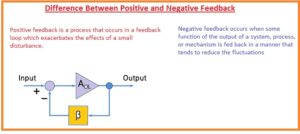Hello, friends welcome to the new post. In this post, we will have a detailed look at Difference Between Positive and Negative Feedback. The feedback is the regulatory system that used to send the output back to the input. The output signal can be in form of current or voltage according to the function of the system uses.

Feedback is divided in two sub-parts first one is positive and the second one is negative that employed in control theory. The basic comparison among them is that for positive feedback the working or effective signal is the summation of real connected input and signals coming from the output. While in the case of negative feedback real signals and feedback signals are different of one another. So let’s get started with.

## Difference Between Positive and Negative Feedback

Positive Feedback

• In this type of feedback, the relationship exists between the processing rate and concentration.
• It also called regenerative feedback in which the input or fed signal and output signal added to provides a strong signal at the input.
• There is a similar phase exists in the input and output signal in case of positive feedback.
• This system provides features to enhance the net gain os system that using it.
• It operates through getting output through the system regenerated then link it to the input supply.
• In a different biological procedure that is output gets through the reaction when it operates as a catalyst.
• The circuit-like RC oscillator, wein bridge oscillators used this feedback system.
• It also called the positive loop gain about the enclosed loop having cause and fact.
• Its configuration is such that it lies with the in-phase arrangements to the input that means it creates the input to a larger value.
• Positive feedback is employed in digital electronics to cause the voltage to change the condition from one to zero.
• There is a certain type of positive feedback that is not good for semiconductor junction and can harm it is thermal runaway.
• This system is used in different reactions to rise the value of reaction operationNegative Feedback

• The negative feedback exists when there a difference or one eighty-phase difference among the input and output signal.
• This feedback system is also called degenerative feedback.
• In this system, 2 signals subtracted and the result then provided to the system. There is a decrement in the net gain of the system since output deleted from the input.
• It also employed or its occurrence appears when the multiple of reaction cause the slow speed of reaction in results system goes to equilibrium,
• It helps to stabilize the system and decreases the net gain.
• An example of negative feedback is electronic amplifiers. Negative feedback is working in running the bandwidths and decreasing the distortion.

### What is a Negative Feedback Amplifier

• In this type of amplifier, the part of the output is subtracted from the input that restricts the real signal. The voltage given can enhance the operation of circuitry and decrease the changes in different terms.
• So this configuration is used in a negative feedback operation In the below figure the circuitry of negative feedback can seen here.### Comparison Between Positive and Negative Feedback

What is Positive Feedback

• It is also known as regenerative feedback.
• There is the in-phase relationship between input and output.
• It increases the net gain of the system.
• There is the use of oscillators.

What is Negative Feedback

• It also called negative feedback
• There is out of phase link between input and output for this system
• Its gain value is less.
• Its stability is higher than positive feedback.

That is a detailed post about Difference Between Positive and Negative Feedback if you has any further query ask in the comments. see you in next post.